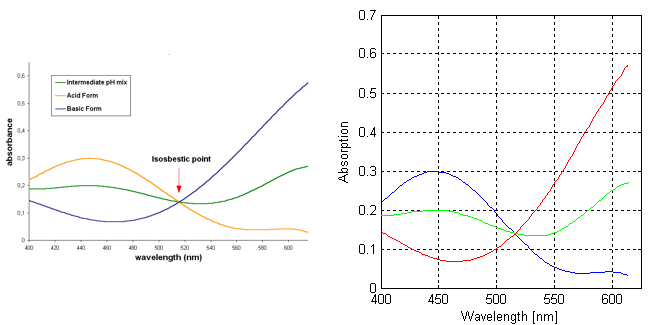The numerical data from graphs digitized with Dagra can be loaded into Matlab using our Matlab function, the system clipboard or a text file.

The easiest method is using the `LoadDagra.m` function because you don’t need to create a separate file or fill up Matlab’s command buffer with the imported data. The options are:

1. The `LoadDagra.m` function for Matlab. This function loads Dagra documents into a structure.
2. Digitized data can also be copied onto the clipboard (Edit→Copy Data… in Dagra) and pasted into the command line window, or
3. exported into a text file (File→Export Data… in Dagra) and imported using Matlab’s dlmread function.

## Setup

`LoadDagra.m` must be on Matlab’s search path before it can be used. The function is installed in the Dagra application directory in the folder `Components\Matlab\`.

If Dagra was installed in the default location, then the command to include it in the search path is:

`LoadDagra.m` takes a single argument, the path to the Dagra document to load. Only the filename is needed if the file is in the current directory. It returns a structure containing fields for each series in the Dagra document:

Spaces in the series name are replaced by underscore characters to form valid field names.

## The Series Structure

Each series structure contains two fields: x and y. Each is a vector containing the exported data. These vectors will always be the same length.

Note: if you are using an expired trial version of Dagra, the exported data contains random noise. Purchase a Dagra license to recover the original high-precision data.

## Plotting the Data

This Matlab code creates a plot showing the graphical source image on the left and data digitized with Dagra on the right:The spectrum of Bromocresol green from Wikipedia (left) and the digitized data plotted in Matlab (right).

## Interpolating the Data

Dagra exports sufficient data to accurately reconstruct the curves. So intermediate values can be obtained with linear interpolation using Matlab’s `interp1` function.

To estimate the absorbance of the acid form of Bromocresol green at 450, 515 and 570 nm, for example:

Not readable? Change text.